# 1. 打印到控制台输出

# How to Print to Console in Python
print("Hello World! Welcome to Python Examples.")
print(10)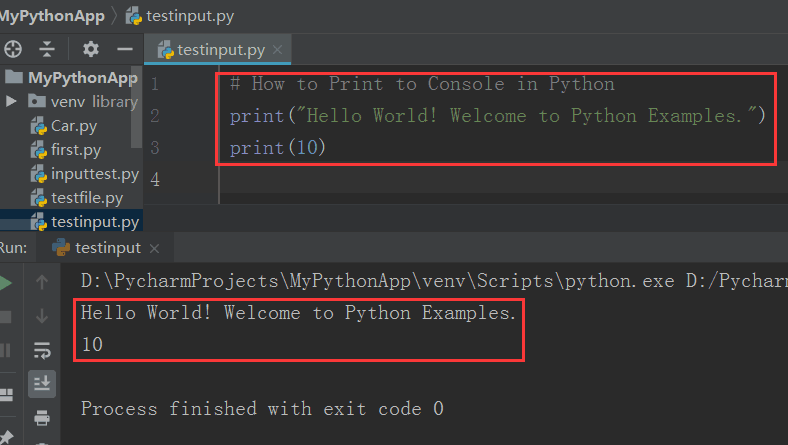## 1.1. 打印字符串到控制台

# Print String to Console
print("Hello World!")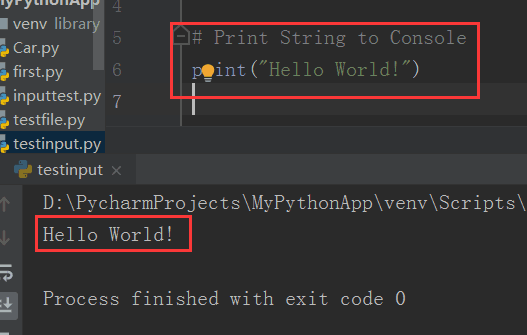## 1.2. 打印数字到控制台

# Print number to console
print(526)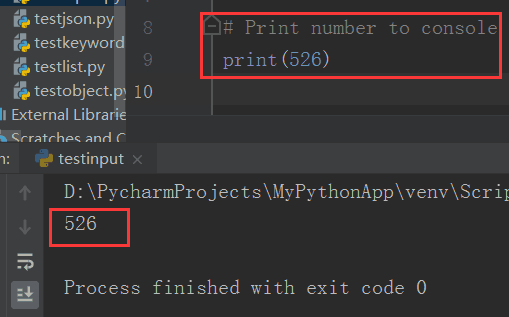## 1.3. 打印变量到控制台

# Print Variable to Console
x = "pi is"
y = 3.14
print(x, y)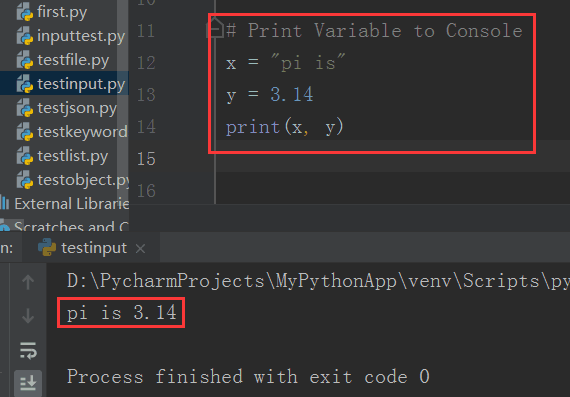## 1.4. 以特定分隔符进行打印

# Python Print with a Specific Separator
x = "pi is"
y = 3.14
print(x, y, sep=" : ")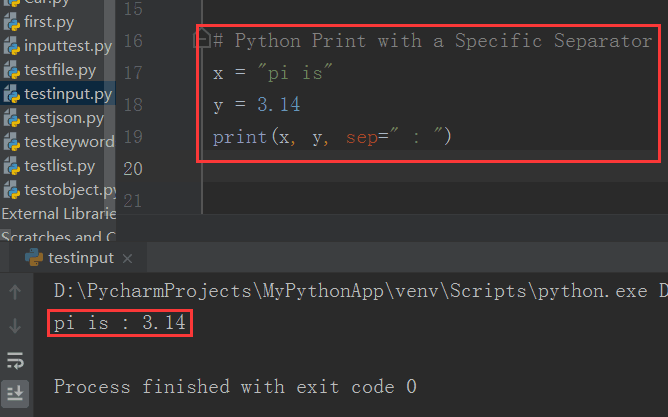## 1.5. 以特定结尾进行打印

# Python Print with a Specific End
print("Hello", end="-")
print("World", end=".\n")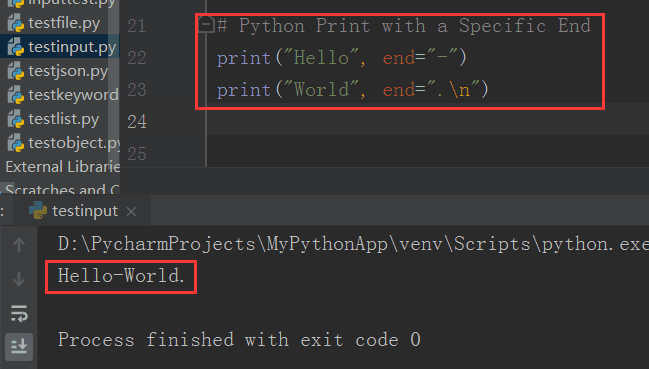# 2. 控制台读取数字

# Read Number from Console
n = int(input("Type a number: "))
print(n)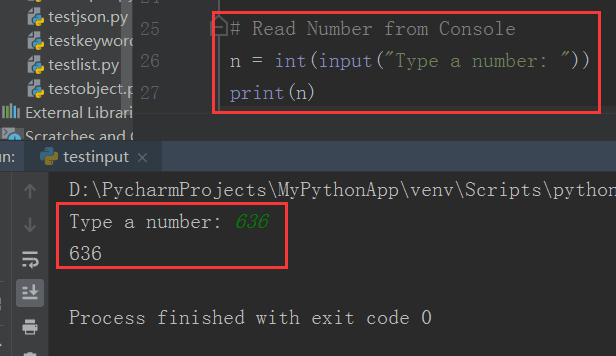# Check if it is a number by performing some arithmetic operations
n = int(input("Type a number: "))
print(n)
print(n + 7)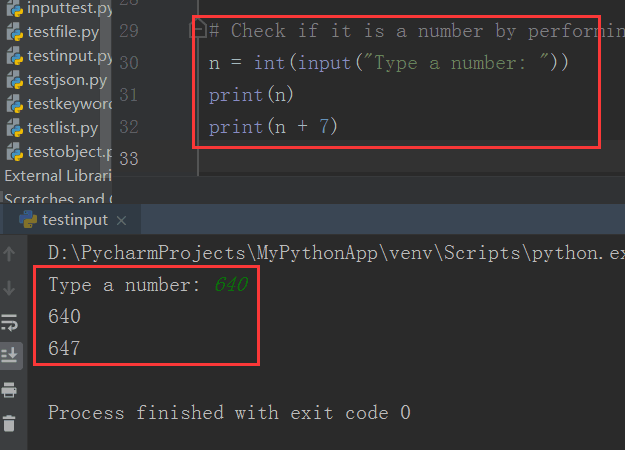# 3. 控制台读取字符串

# Read String from Console
inputStr = input("Enter a string: ")
print(inputStr)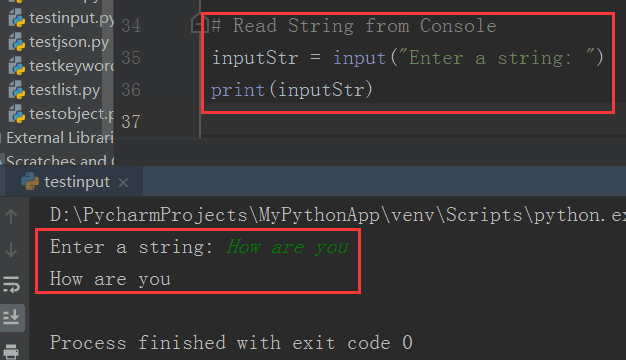input() 函数将返回你在控制台输入的字符串。

# 4. 多次打印之间不换行

print() 函数默认使用换行符作为打印的结束符，要在 Python 中的多次打印之间不换行的话，你可以对 print() 函数的 end=’’ 参数进行设置。

# Print without new line
print("Hello", end='')
print(" World.", end='')
print(" Welcome to", end='')
print(" defonds.net")# 参考资料04-27269
05-19252
08-221502
12-0818万+
01-26315
08-0449
04-28830
08-152485
06-2075